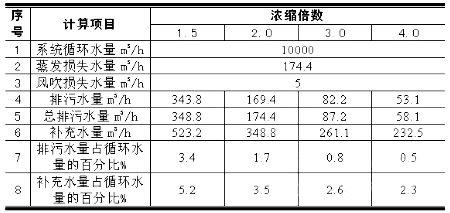# 三缘玻璃钢告诉你：冷却塔的补水问题

（一）、冷却水的补水问题

Qm=Qe+ Qw+Qb

(1) 蒸发损失
Qe= (0.001+0.00002θ) Δt Q (1)

(2) 风吹损失水量

Qw=(0.2%～0.3%)Q (2)
(3) 排污和渗漏损失

N =Cr/Cm

QmCm= (Qw+Qb)Cr
N =Cr/Cm=Qm/(Qw+Qb)=( Qe+ Qw+Qb)/( Qw+Qb) (3)
Qm= QeN/(N 一1)

1、 蒸发损失水量：初步确定冷却塔的补充水量，可按下式计算
Qe=(0.001+0.00002X) t Q=KtQ
Qe---蒸发损失水量 ; t---冷却塔进出水温度差; Q---循环水量;X---外气湿球温度(28.6度)
2、吹损失水量：为(0.2%～0.3%)Q=Qw
3、排污损失水量：Qb

Qm=(0.001+0.00002×28.6)×5 Q×3/(3-1)=0.012Q=1.2%Q=6.8m3/h。（二）、冷却塔的工作过程：

2017-2020版权所有：成都三缘玻璃钢有限责任公司 总经理电话：13908023828 地址：成都市郫都区团结镇平安村八队208号 网址：www.cdsyblg.cn 蜀ICP备05017368号